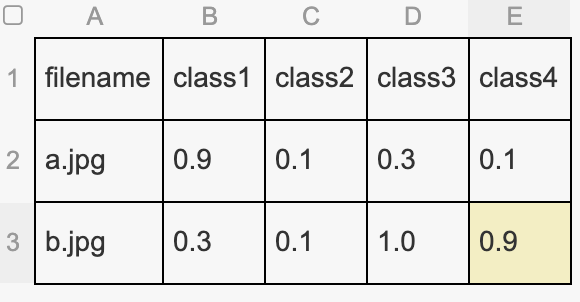Soft labels for Multi-label problems

(Arkar Aung) #1

Is there a way to feed in soft labels for multi-label problems? Normally, what we would do is have delimited labels in csv file and then `ImageItemList` will take care of everything. But that is assuming that every label will be converted to `1`. This is what traditional training looks like. But imagine the case where the training labels are not gold-standard and thus the values associated with the labels are probability values (soft labels). My goal is to have soft labels (like 0.8, 0.5, depending on how unsure the ground truth label is) for multiple labels and train the network with those multiple soft labels. The intuition is to backpropagate lesser for those labels with smaller probability values (and thus making only smaller adjustments to weights for those labels where the ground truth is not very sure of the actual class).

Example with plant dataset:
https://docs.fast.ai/tutorial.data.html#A-multilabel-problem

Here the labels such as, partly_cloudy, primary for a particular image will finally be converted to numerical values such as `[0 1 0 0 0 1]` (assuming that the places of 1’s correspond to the class partly_cloudy and primary respectively and there are a total of 6 labels). But, for example, if we are not sure about partly_cloudy and we are only 70% confident, the soft label scheme would be something like `[0 0.7 0 0 0 1]` for training label.

0 Likes

#2

If they are one-hot (not really ones I understand) encoded, you can use the data block API and pass the option encoded=True (not sure of the name) at your label call. This should work.

0 Likes

(Arkar Aung) #3

Thanks for your reply @sgugger. Are you talking about `one_hot` in `MultiCategoryList`? I cannot find `encoded` that you mentioned.

0 Likes

#4

Yes, that one! Sorry I didn’t remember the name properly0 Likes

(Arkar Aung) #5

Thanks a lot for you time @sgugger. I tried to figure out what I wanted to do. But I am having some problems understanding how to plug in MultiCategoryList with ImageItemList.

What I have right now is a dataframe such as:Essentially, the network would have four output nodes with sigmoid activation units and the training labels are probability values.

I want to pass those classes with MultiCategoryList.

What I am currently doing is:

``````data_src = (ImageItemList.from_df(df=df, path=parent_path, folder='train')
.label_from_df(cols=['class1','class2','class3','class4'], label_cls=MultiCategoryList))
``````

How can I connect this up with MultiCategoryList where I would say `one_hot` to be `True`?

1 Like

(Arkar Aung) #6

What I found out was that if I treat my lables as `FloatList` rather than `MultiCategoryList`, I can at least create a `DataBunch`.

One more thing which makes me wonder is: Are the labels of `MultiCategoryList` essentially similar to `FloatList` with values being 1.0 for correct classes and 0.0 for incorrect classes?

0 Likes

#7

``````data_src = (ImageItemList.from_df(df=df, path=parent_path, folder='train')
.label_from_df(cols=['class1','class2','class3','class4'], label_cls=MultiCategoryList, one_hot=True))
``````

and don’t forget to split before.

Using `FloatList` will also work, but it will make the loss function of your model MSE, so you will need to adjust that manually.

0 Likes

(Arkar Aung) #8

Oh I see. Thanks a lot for pointing out that the loss will be MSE with `FloatList`.

When I tried what you mentioned, `DataBunch` shows something like this for labels:

``````y: MultiCategoryList
,,,,
``````

I thought it didn’t work. But when I checked the batch of y values in `train_dl`, floating point values are indeed loaded.

0 Likes

#9

You also have to pass `classes` when using one-hot encoded labels like this, because the API can’t guess them. That’s why your visualization is weird. It will still be a bit weird though, as your labels are supposed to be 0. or 1. and they won’t.

0 Likes

(Arkar Aung) #10

Thanks again for pointing that out. I thought specifying `cols` was enough.

So, it should be something like this. Is it correct?

``````data_src = (ImageItemList.from_df(df=df, path=parent_path, folder='train')
.label_from_df(cols=['class1','class2','class3','class4'], label_cls=MultiCategoryList, one_hot=True, classes=['class1','class2','class3','class4']))
``````

`MultiCategoryList` should pick up that extra `kwarg`, `classes` from `label_from_df`, is that correct?

0 Likes

#11

It should yes, though your are right,specifying cols should be enough. Let me know if this new line changes anything.

0 Likes

(Arkar Aung) #12

As far as I can see, the addition of `classes` parameter does not change anything. As expected, the output for labels with probabilities values are not show (as you have mentioned).

0 Likes

(Peter Zacho) #13

Did you manage to solve this problem? I’m really interested as I’ve got a similar data set. Any references to working code is much appreciated.

0 Likes

(Arkar Aung) #15

Yes, I solved by specifying `MultiCategoryList` for `label_cls` and `one_hot` as True. Also add `cols` parameter.

0 Likes